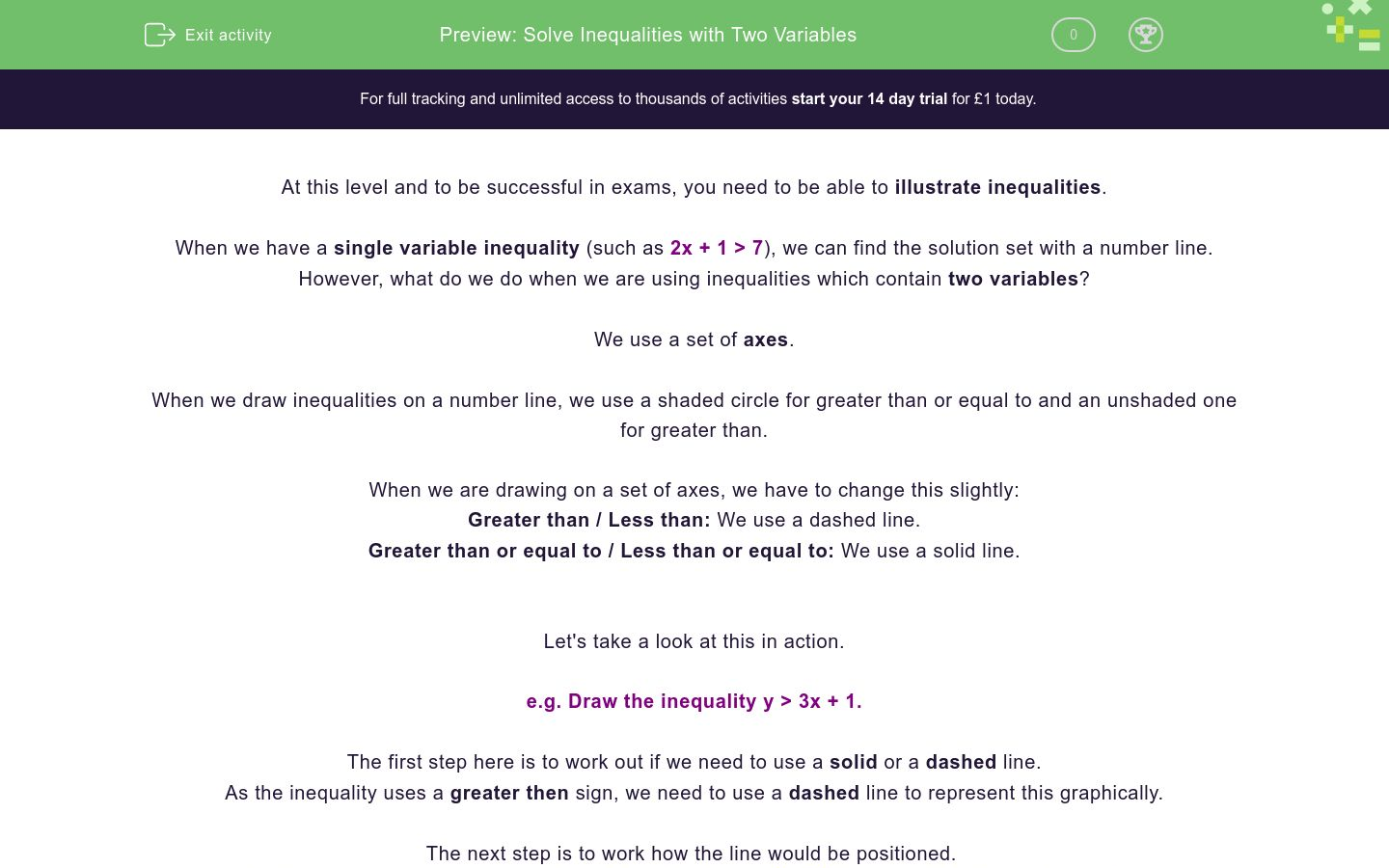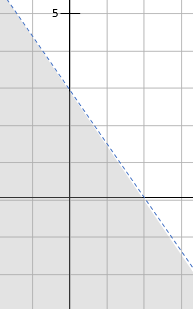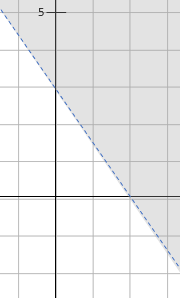# Solve Inequalities with Two Variables

In this worksheet, students will learn how to draw inequalities as graphs, identify the correct graphical representation of an inequality and solve problems relating to these.Key stage:  KS 4

GCSE Subjects:   Maths

GCSE Boards:   AQA, Eduqas, OCR, Pearson Edexcel

Curriculum topic:   Algebra

Curriculum subtopic:   Solving Equations and Inequalities, Algebraic Inequalities

Difficulty level:### QUESTION 1 of 10

At this level and to be successful in exams, you need to be able to illustrate inequalities.

When we have a single variable inequality (such as 2x + 1 > 7), we can find the solution set with a number line.

However, what do we do when we are using inequalities which contain two variables?

We use a set of axes.

When we draw inequalities on a number line, we use a shaded circle for greater than or equal to and an unshaded one for greater than.

When we are drawing on a set of axes, we have to change this slightly:

Greater than / Less than: We use a dashed line.

Greater than or equal to / Less than or equal to: We use a solid line.

Let's take a look at this in action.

e.g. Draw the inequality y > 3x + 1.

The first step here is to work out if we need to use a solid or a dashed line.

As the inequality uses a greater then sign, we need to use a dashed line to represent this graphically.

The next step is to work how the line would be positioned.

To do this, we ignore the inequality and draw the line y = 3x + 1 (using a dashed line of course!):Once we have drawn this line, we need to decide which side to shade to illustrate the inequality.

To do this, we pick a point, plug this into the inequality and see if the point satisfies the inequality.

We think 0,0 is the best one to use but that's just our choice!

We know at (0, 0), x = 0 and y = 0:

y > 3x + 1

0 > 3(0) + 1

0 > 1

We can see very clearly that this is not true, so we shade above the line.

Here is our final graph of this inequality:e.g. Draw the inequality 5x + 2y ≤ 10.

Once again, we pick our line type first.

As this sign is less than or equal to, we need to use a solid line:Once again, we need to test to see which side we need to shade.

We're going to use (0, 0) again:

5x + 2y ≤ 10

5(0)+ 2(0) ≤ 10

0 ≤ 10

Because this statement is true, we know that this is the side of the line we need to shade, like this:Let's try one more of a slightly different type.

e.g. Draw the inequality -2 < x ≤ 1.

It is really common with this style of question that we have to draw single variable inequalities on a set of axes.

To draw this, we have to look at the inequality as two lines:

One line needs to be drawn at x = -2 and needs to be dashed (due to the use of the < sign).

The other line needs to be drawn at x = 1 and needs to be solid (due to the use of the ≤ sign).

If we draw this on a set of axes, we get:We don’t need to test this one, as the inequality is already quite clear.

We are looking for the points between -2 and 1 so our shading on the graph needs to look this this:In this activity, we will find the correct graphs to illustrate inequalities and solve problems using this information.

You will not have to draw any graphs yourself in this activity, but it is important that you understand the rules for doing so.

After you have completed the activity, you may want to go back through each question and practise drawing graphs for any inequalities which have not been graphically represented.

Read the statement below and add a word in the blank to ensure it is accurate.

Type words in each blank to correctly complete the statements below.

Which of the graphs below correctly shows the equation y = 2x + 1?Which of the graphs below correctly shows the inequality y < 2x + 1?Match each inequality below with its graphical function.

## Column B

');" onmouseout="tooltip.hide();">

[Image_Link]/userfiles/image/Active%20Imag...

y > x + 4

');" onmouseout="tooltip.hide();">

[Image_Link]/userfiles/image/Active%20Imag...

y < 2x – 3

');" onmouseout="tooltip.hide();">

[Image_Link]/userfiles/image/Active%20Imag...

y > 3x + 1

Match each inequality below with its graphical function.

## Column B

y > x

');" onmouseout="tooltip.hide();">

[Image_Link]/userfiles/image/Active%20Imag...

y < x

');" onmouseout="tooltip.hide();">

[Image_Link]/userfiles/image/Active%20Imag...

y ≥ x

');" onmouseout="tooltip.hide();">

[Image_Link]/userfiles/image/Active%20Imag...

y ≤ x

');" onmouseout="tooltip.hide();">

[Image_Link]/userfiles/image/Active%20Imag...

Which of the coordinates below satisfy the inequality y > 4x – 5?

(0, 0)

(2, 3)

(0, 4)

(1, -1)

Which of the coordinates below satisfy the inequality 2x + 3y ≤ 6?

(0, 0)

(2, 3)

(0, 4)

(1, -1)

Which of the graphs below correctly illustrates -4 < x ≤ 5?Which of the graphs below correctly illustrates 3x + 2y < 6?• Question 1

Read the statement below and add a word in the blank to ensure it is accurate.

EDDIE SAYS
All inequalities we have to draw at GCSE are linear, this means that you will not be asked to show an inequality which does not show a straight line. Therefore, you will always be drawing a line graph if you are asked to draw or choose a graphical inequality. This is a good tip to remember!
• Question 2

Type words in each blank to correctly complete the statements below.

EDDIE SAYS
If an inequality uses a greater than or equal to sign (≥), we show that possible solutions can lie on the line. We show this by using a solid line. If the line we use is dashed, this means that solutions cannot be on the line which matches with the greater than sign (>). That's quite a sensible approach, isn't it?
• Question 3

Which of the graphs below correctly shows the equation y = 2x + 1?EDDIE SAYS
Its really important in these questions that you read everything closely. This question doesn’t ask for an inequality, it asks for a linear equation. This means there will be no shading and only a solid line. We need to remember to always draw linear equations with a solid line.
• Question 4

Which of the graphs below correctly shows the inequality y < 2x + 1?EDDIE SAYS
This time we are looking for an equation, so we will see shading and either a solid or dashed line. As the inequality uses the less than symbol (<), we know we are looking for a dashed line. To work out which side we need to shade, we must test a point. If we use (0, 0) then we need to substitute x = 0 and y = 0 into this inequality: y < 2x + 1 0 < 2(0) + 1 0 < 1 Because this statement is true, this means that the point (0,0) is on the side of the line that satisfies the inequality so we shade here.
• Question 5

Match each inequality below with its graphical function.

## Column B

');" onmouseout="tooltip.hide();">

[Image_Link]/userfiles/im...

y > 3x + 1

');" onmouseout="tooltip.hide();">

[Image_Link]/userfiles/im...

y < 2x – 3

');" onmouseout="tooltip.hide();">

[Image_Link]/userfiles/im...

y > x + 4
EDDIE SAYS
Do we even need to think about the inequalities here? Let’s remember that when an equation in the form y = mx + c is graphed, the value of c is the point where the line intercepts the y axis. We can use this fact to match the graphs and inequalities much easier and more quickly.
• Question 6

Match each inequality below with its graphical function.

## Column B

y > x

');" onmouseout="tooltip.hide();">

[Image_Link]/userfiles/im...

y < x

');" onmouseout="tooltip.hide();">

[Image_Link]/userfiles/im...

y ≥ x

');" onmouseout="tooltip.hide();">

[Image_Link]/userfiles/im...

y ≤ x

');" onmouseout="tooltip.hide();">

[Image_Link]/userfiles/im...

-->
EDDIE SAYS
This question is all about the shading and the lines. Remember that the lines have different meanings. A solid line represents the < or > signs, whilst a dashed line represents the ≤ or ≥ signs. So we have narrowed each inequality down to two possible options. If we test a point on each graph, we will see that greater than inequalities (using > or ≥) will be shaded above the line. The less than inequalities (< or ≤) will be shaded below the line. Did you match those correctly?
• Question 7

Which of the coordinates below satisfy the inequality y > 4x – 5?

(0, 0)
(0, 4)
EDDIE SAYS
To test each of these, we just need to bang the numbers in and see if the statements we reach are true or false. For example, using (2, 3): y > 4x – 5 3 > 4(2) – 5 3 > 3 This statement doesn’t work because 3 isn’t greater than 3, so we know that (2, 3) does not satisfy this inequality.
• Question 8

Which of the coordinates below satisfy the inequality 2x + 3y ≤ 6?

(0, 0)
(1, -1)
EDDIE SAYS
This is another question where we plug in the options. If our answer is true, the coordinates satisfy the inequality; if is false, then they do not. e.g. (2, 3) 2(2) + 3(3) ≤ 6 4 + 9 ≤ 6 13 ≤ 6 Is 6 greater than or equal to 13? As this statement is not true, this point does not satisfy the inequality.
• Question 9

Which of the graphs below correctly illustrates -4 < x ≤ 5?EDDIE SAYS
The most common mistake here is to get the lines the wrong way round. If the inequality involves x, the lines will be vertical. If it involves y, they will be horizontal. So we are looking for the graph with two vertical lines at x = -4 and x = 5, with the area between them shaded. Did you spot that?
• Question 10

Which of the graphs below correctly illustrates 3x + 2y < 6?EDDIE SAYS
Both graphs show the correct line for the equation 3x + 2y = 6, and they both used the dashed line to match the use of the < sign. So we need to work out which area needs to be shaded to find our correct answer. The easiest way to test this is to test a point and see if it creates a true or false statement. If we use (0, 0): 3x + 2y < 6 3(0) + 2(0) < 6 0 < 6 This statement is true so we know that (0, 0) needs to be on the shaded side of the line. Congratulations for completing this activity! For some extra practise, you may want to go back through this activity and draw graphs to represent any inequalities which do not currently have a graph shown.
---- OR ----

Sign up for a £1 trial so you can track and measure your child's progress on this activity.

### What is EdPlace?

We're your National Curriculum aligned online education content provider helping each child succeed in English, maths and science from year 1 to GCSE. With an EdPlace account you’ll be able to track and measure progress, helping each child achieve their best. We build confidence and attainment by personalising each child’s learning at a level that suits them.

Get started